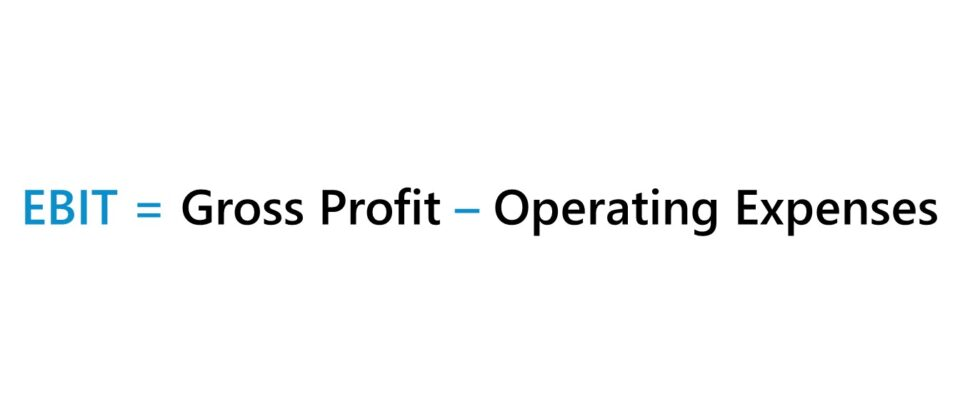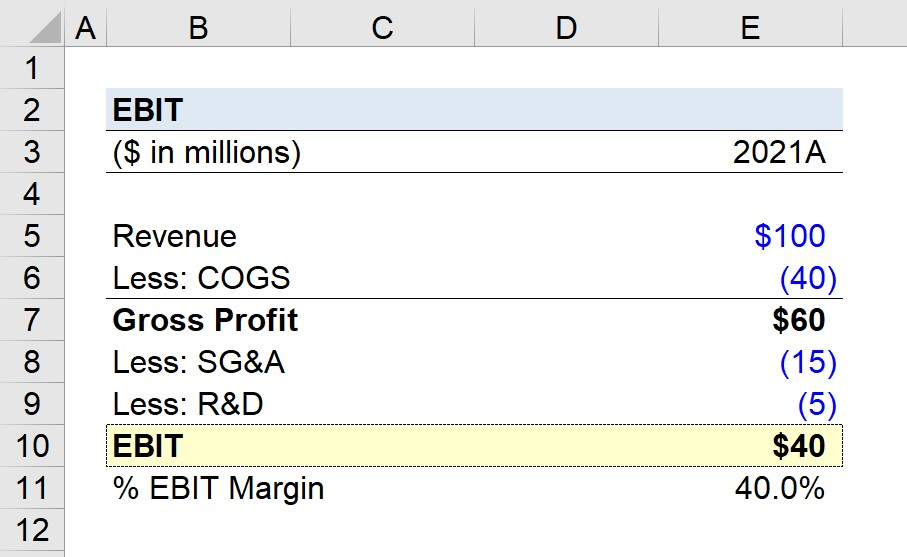Welcome to Wall Street Prep! Use code at checkout for 15% off.# EBIT

Guide to Understanding Earnings Before Interest and Taxes (EBIT)• EBIT is a company’s operating profitability in a given period once COGS and operating expenses are deducted.
• The formula for calculating EBIT is gross profit minus operating expenses (COGS, SG&A, R&D, S&M).
• EBIT is a commonly used profitability metric for relative valuation and peer comparisons because it is unaffected by discretionary decisions such as debt financing, non-core income sources, one-time corporate decisions, and taxes.
• EBIT is different from EBITDA in that the metric abides by GAAP reporting standards and thus appears on the income statement, while EBITDA is a non-GAAP metric that adds back depreciation and amortization, among other adjustments.

## How to Calculate EBIT?

EBIT, or “operating income”, measures the operating profitability of a company in a specific period, with all core operating costs, i.e. COGS and operating expenses, deducted from revenue.

On the income statement, operating income takes into account the following line items:

• RevenueThe net sales generated throughout the period.
• Cost of Goods Sold (COGS)The direct costs incurred in the period (i.e. tied to how revenue is produced).
• Operating Expenses (OpEx)The indirect costs incurred in the period (i.e. not directly tied to revenue generation but integral to business operations).

Understanding the meaning of revenue (the “top line) should be relatively straightforward because it represents the net sales earned by a company from its operations, i.e. selling products and services to customers.

As for cost of goods sold (COGS) and operating expenses (OpEx), the distinction was mentioned earlier, where the former consists of direct costs while the latter comprises indirect costs.

But the matter of importance here is that COGS and operating expenses (OpEx), such as selling, general and administrative (SG&A), research and development (R&D) and sales and marketing (S&M) are incurred as part of the company’s core operations.

In other words, all expenses above the operating income line item are deemed “operating costs” while those below the line such as interest expense and taxes are “non-operating costs”.

## EBIT Formula

Written out, the formula for calculating a company’s operating income (EBIT) is as follows:

EBIT = Gross ProfitOperating Expenses
• Gross Profit = Revenue – Cost of Goods Sold (COGS)
• Operating Expenses = Σ Indirect Operating Costs

A company’s revenue is the starting line item on the income statement, while COGS is the first deduction from the “top line”, resulting in a company’s gross profit.

From a company’s gross profit, the next step is to subtract its operating expenses to arrive at the operating income line item.

The composition of a company’s operating expenses will vary by the specific circumstances (e.g. industry, cost structure), but the more common operating expenses are the following:

## Quick EBIT Calculation Example

For example, imagine that a publicly traded company in 2021 reported the following financials:

• Net Revenue = \$25 million
• Cost of Goods Sold (COGS) = –\$10 million
• Operating Expenses = –\$5 million

The gross profit is equal to \$15 million, from which we deduct \$5 million in OpEx to calculate operating income.

• Gross Profit = \$25 million – \$10 million = \$15 million
• Operating Income = \$15 million – \$5 million = \$10 million

## EBIT Margin Calculation Example

Continuing off our previous example, we can divide our company’s operating income by its revenue to calculate the operating margin.

EBIT Margin (%) = EBIT ÷ Revenue

Since the operating income is \$10 million, we’ll divide that profit metric by our revenue of \$25 million.

Since comparisons of standalone operating profit amounts are not meaningful, standardization is required, which is the purpose of multiples.

In our example, the operating margin is 40% — which means that for each dollar of revenue generated, \$0.40 is retained and available for non-operating expenses.

The 40% margin can be compared against that of comparable peers, as well as with historical margins, to evaluate the financial performance of the company in question.

## What is a Good EBIT?

The previous calculation of the EBIT margin leads to our next point.

Other than EBITDA, EBIT is likely the most commonly used profitability metric for relative valuation and peer comparisons.

Why? EBIT is unaffected by discretionary decisions, such as:

All relative valuation is skewed to some extent, but by using an unlevered metric like EBIT, a significant amount of flaws can be avoided.

It is important to note that one of the primary objectives of relative valuation is to compare the core operations of comparable companies, as opposed to the non-core operations.

For example, let’s say that there are two companies with net margins of 40% and 20%.

However, the operating margins of the two companies could be much closer, as the cause of the 20% differential might be related to capital structure decisions — i.e. the company with 20% net margins might have undergone an LBO, so incurs substantial interest expense each period.

By comparing the operating margin, these non-core differences are intentionally neglected to facilitate more meaningful comparisons among peer groups.

## What is the Difference Between EBIT vs. EBITDA?

To reiterate from earlier, EBIT and EBITDA are two of the most frequently used metrics for peer comparisons.

Like EBIT, EBITDA removes the effect of capital structure decisions and taxes — however, depreciation & amortization is added back since they represent non-cash charges (as well as sometimes stock-based compensation).

EBIT = Gross Profit Operating Expenses
EBITDA = EBIT + Depreciation + Amortization

Both of the profit metrics are informative measures of a company’s profitability and operational performance. Hence, both metrics appear in the majority of comps sheets.

EBITDA is typically a larger amount than operating income because of the non-cash add-backs, with the difference becoming more apparent for capital-intensive industries (i.e. large number of fixed assets + depreciation expenses), which is an area of frequent criticism.

• GAAP: Another distinction between the two is that operating income is a GAAP metric and appears on the income statement.
• Non-GAAP: On the other hand, EBITDA is a non-GAAP metric, and the reconciliation is typically shown separately in SEC filings, as well as used in investor presentations and by equity analysts.

## EBIT Calculator

We’ll now move to a modeling exercise, which you can access by filling out the form below.Submitting...

## 1. Income Statement Assumptions

Suppose we’re given the 2021 income statement of a company and tasked with calculating its EBIT.

Starting from the company’s \$100 million in revenue, the first step is to deduct COGS, which is stated as \$40 million.

• Revenue = \$100 million
• COGS = \$40 million

## 2. Gross Profit Calculation

The gross profit is equal to \$60 million, which we calculated by subtracting COGS from revenue.

• Gross Profit = \$100 million – \$40 million = \$60 million

Given the \$60 million in gross profit, the gross margin comes out to 60%, i.e. for each dollar of revenue generated, \$0.60 is kept as gross profit after deducting COGS.

## 3. EBIT Calculation Example

From gross profit, we must now subtract the company’s operating expenses, wherein there are two types recorded.

1. SG&A Expense = \$15 million
2. R&D Expense = \$5 million

The total operating expense amounts to \$20 million, which we’ll use to reduce gross profit and arrive at an EBIT of \$40 million for our hypothetical company.

• Total Operating Expenses = \$15 million + \$5 million = \$20 million
• EBIT = \$60 million – \$20 million = \$40 million

## 4. Operating Margin Analysis Example

In closing, we’ll divide our company’s operating income by its revenue in the corresponding period to arrive at an operating margin of 40% to standardize the metric for purposes of comparability.

• Operating Margin (%) = \$40 million ÷ \$100 million = 40%Step-by-Step Online Course

### Everything You Need To Master Financial Modeling

Enroll in The Premium Package: Learn Financial Statement Modeling, DCF, M&A, LBO and Comps. The same training program used at top investment banks.Inline FeedbacksLearn Financial Modeling Online

Everything you need to master financial and valuation modeling: 3-Statement Modeling, DCF, Comps, M&A and LBO.

X

The Wall Street Prep Quicklesson Series

7 Free Financial Modeling Lessons

Get instant access to video lessons taught by experienced investment bankers. Learn financial statement modeling, DCF, M&A, LBO, Comps and Excel shortcuts.